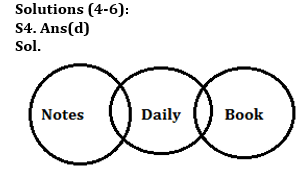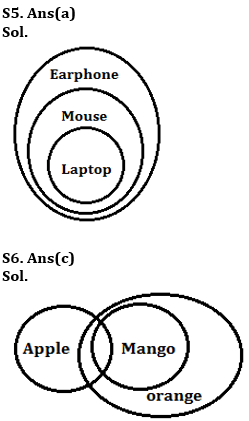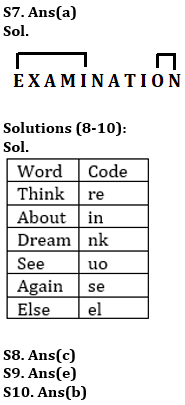Latest Banking jobs   »

# Reasoning Quizzes Quiz For IBPS RRB PO/Clerk Prelims 2023-03rd June

Directions (1-3): In these questions, relationship between different elements is shown in the statements. The statements are followed by conclusions. Study the conclusions based on the given statements and select the appropriate answer:
(a) If only conclusion I follows
(b) If only conclusion II follows
(c) If either conclusion I or II follows
(d) If neither conclusion I nor II follows
(e) If both conclusions I and II follow

Q1. Statements: A = G ≥ T > I > W ≤ O ≥ B, Y ≥ T > U
Conclusions: I. Y > W II. O > A

Q2. Statements: A ≤ Q = Z = M ≤ B ≥ P = Y ≤ F
Conclusions: I. B > A II. B = A

Q3. Statements: Q < G > J = Z ≤ L > M = V ≤ I > P
Conclusions: I. I ≥ J II. J > I

Directions (4-6): In each of the questions below, some statements are given followed by two conclusions. You have to take the given statements to be true even if they seem to be at variance with commonly known facts. Read all the conclusions and then decide which of the given conclusions logically follows from the given statements disregarding commonly known facts.
(a) If only conclusion I follows
(b) If only conclusion II follows
(c) If either conclusion I or II follows
(d) If neither conclusion I nor II follows
(e) If both conclusions I and II follow

Q4. Statements: Some Notes are Daily.
Some Daily are Book
Conclusion I: No Notes is book
II: All Book are Notes

Q5. Statements: All laptop are Mouse.
All Mouse are Earphone.
Conclusion I: Some Mouse are laptop
II: All Earphone are laptop

Q6. Statements: Some Apple are Mango.
All Mango are Orange
Conclusion I: Some Apple are not Orange
II: All Apple are Orange

Q7. How many pairs of letters are in the word ‘EXAMINATION’, each of which have as many letters between them as they have between them in the English alphabet?
(a) Two
(b) Three
(c) Four
(d) One
(e) None of these

Directions (8-10): Study the information carefully and answer the questions given below.
In the coded language,
“Think About Dream” is coded as “nk in re”
“See Again About” is coded as “se in uo”
“Dream See Else” is coded as “nk uo el”

Q8. What is the code for the word “Again”?
(a) in
(b) nk
(c) se
(d) uo
(e) None of these

Q9. What is the code for the word “Dream Else”?
(a) re uo
(b) uo in
(c) nk se
(d) re nk
(e) None of these

Q10. What is the word for the code “uo”?
(a) Else
(b) See
(c) Think
(d) Again
(e) Either (a) or (d)

Solutions

Solutions (1-3):
S1. Ans(a)
Sol. I. Y > W(True) II. O > A(False)

S2. Ans(c)
Sol. I. B > A(False) II. B=A(False)

S3. Ans(c)
Sol. I. I ≥ J(False) II. J > I(False)## FAQs

### What is the selection process of IBPS RRP PO?

There are 4 sections in the IRDAI assistant manager online preliminary exam. They are Reasoning, English Language, General awareness, Quantitative aptitude.

#### Congratulations!Union Budget 2023-24: Free PDF